• python判断三角形
千次阅读
2021-11-20 14:30:47
a,b,c=map(int ,input("请输入三个整数，以空格隔开：").split())
if a<b+c and b<a+c and c<a+b:
if a==b==c:
print('等边三角形')
elif a==b or a==c or b==c:
if a*a+b*b==c*c or a*a+c*c==b*b or b*b+c*c==a*a:
print('等腰直角三角形')
else:
print('等腰三角形')
elif a*a+b*b==c*c or a*a+c*c==b*b or b*b+c*c==a*a:
print('直角三角形')
else:
print('普通三角形')
else:
print('无法构成三角形')selenium 测试工具 python
更多相关内容
• 主要介绍了Python3如何判断三角形的类型，具有很好的参考价值，希望对大家有所帮助。一起跟随小编过来看看吧
• ## Python判断三角形

千次阅读 2020-12-29 10:51:14
#Copyright (c)2017, 东北大学软件学院学生# All rightsreserved#文件名称：a.py# 作 者：孔云#问题描述：编写程序，从键盘输入三条边，判断是否能够构成一个三角形。#问题分析：组成三角形的条件是任意两边之和大于...

# All rightsreserved

#文件名称：a.py

# 作 者：孔云

#问题描述：编写程序，从键盘输入三条边，判断是否能够构成一个三角形。

#问题分析：组成三角形的条件是任意两边之和大于第三边，如果条件成立，则能构成三角形。条件表达式中的多个条件必须全部成立，条件之间使用and运算符连接。代码如下：

side1=float(input("the first side of triangle:"))

side2=float(input("the second side of triangle:"))

side3=float(input("the thrid side of triangle:"))

if(side1+side2>side3)and(side2+side3>side1)and(side3+side1>side2):

print (side1,side2,side3,"is sides of triangle")

else:

print (side1,side2,side3,"is not sides of triangle")

运行结果如下：注：在这注意的是条件表达式中使用and运算符，而不是&！！！

展开全文• python已知三角形两条边长分别为10，8，请输入第三条边的边长，判断可以围成三角形，如果可以围成三角形判断是等腰三角形还是普通三角形，并输出判断结果，如果不能围成三角形，则输出不能围成三角形python
• 如何利用简单代码实现三角形判断 a,b,c=map(int,input('输入三角形边长：').split(' '))//输入三条边的边长 if(a + b > c and b + c > a and a + c > b)://先判断三角形是否成立，是继续执行以下代码 ...

如何利用简单代码实现三角形的判断

a,b,c=map(int,input('输入三角形边长：').split(' '))//输入三条边的边长
if(a + b > c and b + c > a and a + c > b)://先判断三角形是否成立，是继续执行以下代码
if(a == b ==c)://判断是否为等边三角形
print('等边三角形')
elif (a == b and a!=c)://不满足上述语句，再判断等腰三角形
print('等腰三角形')
elif (a == c and a!=b)://不满足上述语句，再判断等腰三角形
print('等腰三角形')
elif (b == c and b!=a)://不满足上述语句，再判断等腰三角形
print('等腰三角形')
elif (a**2 + b**2 == c**2)://不满足上述语句，再判断直角三角形
print('直角三角形')
elif (b**2 + c**2 == a**2)://不满足上述语句，再判断直角三角形
print('直角三角形')
elif (a**2 + c**2 == b**2)://不满足上述语句，再判断直角三角形
print('直角三角形')
print('一般三角形')不满足上述语句为一般三角形
else://不成立输出不成立
print('该三角形不成立')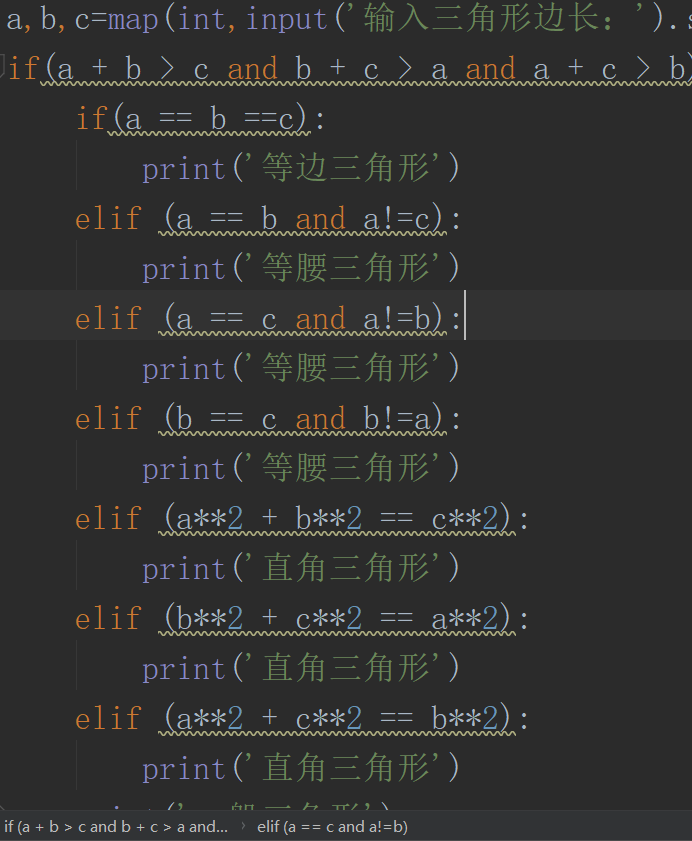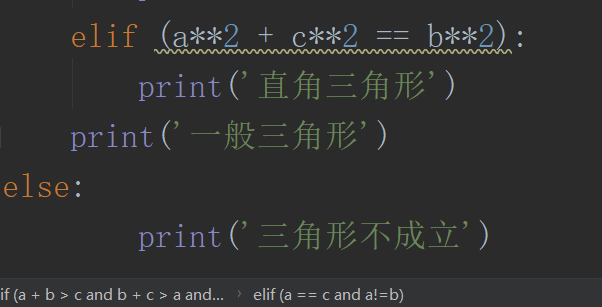本次涉及到if，elseif=elif结构知识

if语句

if<test1>://先进行条件测试

<语句1>

elif<test2>://与test1同层次的多个选择

<语句2>

elif<test3>://与test1同层次的多个选择

<语句3>

.......

else://上述条件测试全为假，则执行else

展开全文python
• PYTHON判断三角形的类型工具/材料PYTHON操作方法01首先打开PYTHON,新建一个空白的PY文档。02这里要判断的三角形类型有三种，不等边三角形，等腰三角形和等边三角形。03先定义三个边都要输入数值。a = int(input(...

用PYTHON判断三角形的类型

工具/材料

PYTHON

操作方法

01

首先打开PYTHON,新建一个空白的PY文档。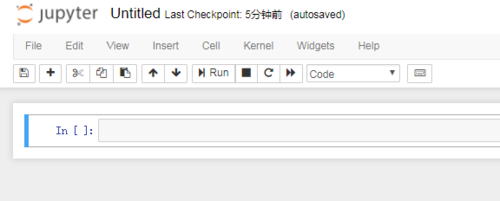02

这里要判断的三角形类型有三种，不等边三角形，等腰三角形和等边三角形。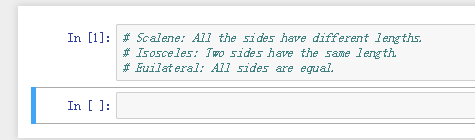03

先定义三个边都要输入数值。

a = int(input("The length of the side a = "))

b = int(input("The length of the side b = "))

c = int(input("The length of the side c = "))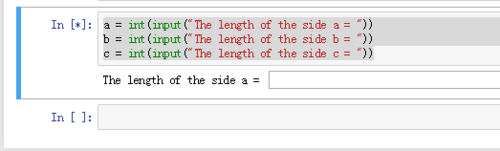04

if a != b and b != c and a != c:

print("This is Scalene")

elif a == b and b == c:

print("This is an Equilateral")

else:

print("This is Isosceles")

定义每种情况下需要打印什么结果出来。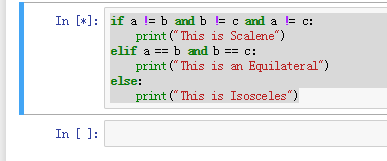05

测试一下不同输入得到的不同结果。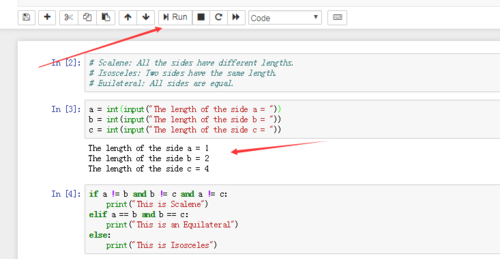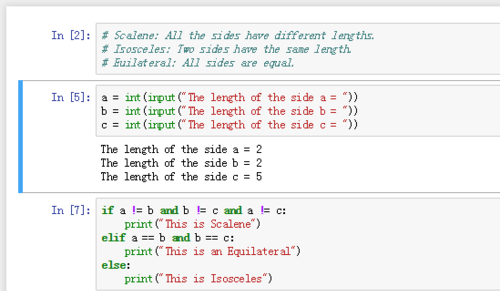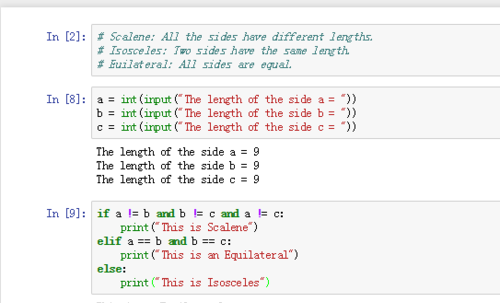06

如果输入不是数值将会报错，因为定义变量的时候已经规定要要输入什么了。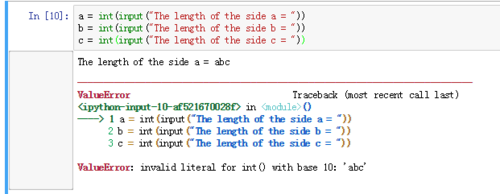好了，以上就是大致内容了，(END)

注意四个空格的间隔

声明：该文观点仅代表作者本人，天晴资讯网系信息发布平台，仅提供信息存储空间服务。

展开全文• a,b,c=map(int ,input().split()) if a<b+c and b<a+c and c<a+b: if a==b==c: print('等边三角形') elif a==b or a==c or b==c: if a*a+b*b==c*c or a*a+c*c==b*b or b*b+c*c==a*a: ...
• 请从标准输入流（控制台）中获取 a、b、c，它们分别代表三角形的三条边长，判断这三条边是否能组成一个三角形，当判断为是时，你应该使用 print 语句输出 Is a triangle，当判断为否时，则使用 print 语句输出 Not a...python
• python实现三角形判断 massage = ''' 已经给出三条边a，b，c,能否构成三角形，如果能构成三角形判断三角形的类型（等边三角形、等腰三角形或普通三角形）。 输入描述: 题目有多组输入数据，每一行输入三个a，b，c...python
• def judge(a,b,c): if a<=0 or b<... print("不构成三角形") elif a==b & b==c & c==a: print("等边三角形") elif a==b or b==c or c==a : print("等腰三角形") else : printpython
• 获取键盘输入的三角形三边,判断是否可以组成三角形,如果能组成三角形,进一步判断是什么三角形(普通、等腰、等边、直角) 分析： 看到这个题目，我们首先应该想到的是用if 条件语句，在分析形成三角形的条件是什么？...python 软件测试
• 假设在平面内，有一个三角形，边长分别为a，b，c，三角形的面积S可由以下公式求得: 而公式里的p为半周长 #（1）输入边长 a = float(input('输入三角形第一边长: ')) b = float(input('输入三角形第二边长: ')) c ...
• 参考方法：# 通过用户输入三角形三边长度，并计算三角形的面积# 已知三角形三边a,b,c，则# (海伦公式)(p=(a+b+c)/2)# S=sqrt[p(p-a)(p-b)(p-c)]# =sqrt[(1/16)(a+b+c)(a+b-c)(a+c-b)(b+c-a)]# =1/4sqrt[(a+b+c)(a+b-...
• #判断构成三角形import mathtrianglea = int(input(“请输入第条边长a”))triangleb = int(input(“请输入第条边长b”))trianglec = int(input(“请输入第条边长c”))if(trianglea > 0 and triangleb > 0 and ...
• 三角形面积 from math import sqr a = float(input('a = ')) b = float(input('b = ')) c = float(input('c = ')) if a + b > c and b + c > a and c + a > b: p = (a + b + c) / 2 area = sqrt(p * (p...
• 主要介绍了Python判断三段线能否构成三角形的代码，具有很好的参考价值，希望对大家有所帮助。一起跟随小编过来看看吧
• python三角形判定怎么做？下面给大家带来具体的例子：相关推荐：《Python视频教程》例子：a = int(input("The length of the side a = "))b = int(input("The length of the side b = "))c = int(input("The length ...
• 主要讲述了python代码判断三角形类型，使用pytest对判断三角形类型代码的单元测试，生成allure的测试报告。pytest python
• python 判断用户输入的三个边是否为三角形，若符合 求其面积python
• A = input("请输入三角形边长A：") B = input("请输入三角形边长B：") C = input("请输入三角形边长C：") # 以下是判断输入值是否为字符，不是字符再继续执行，是字符则结束程序不再执行 if A.isdigit() and B....
• 利用python计算三角形的面积。（1）输入三个数，作为三角形的三个边长，利用海伦公式计算三角形的面积。海伦公式：假设在平面内，有一个三角形，边长分别为 a、b、c，三角形的面积 S可由以下公式求得。S=根号p(p-a)...
• 满意答案hanfangyi2013.09.25采纳率：45%等级：12已帮助：5622人triangle1 = (5, 5, 5)triangle2 = (3, 4, 5)triangle3 = (3, 4, 5)triangle4 = (1, 2, 5)triangle5 = (2, 2.5, 3.9)def is_triangle(sides):a, b, c ...
• 三角形按边为：等边，等腰 等边：三条边相等； 等腰：两条边相等 ...#判断三角形 def triangle(a,b,c): if a>0 and b>0 and c>0: if a+b>c and a+c>b and b+c>a: if a==b and b==c:python
• ## Python编写三角形

千次阅读 2021-10-30 15:58:57
2.第一个for循环判断行数，第二个for循环判断每一行的数字并空一格输出 3.当第二个for循环结束时需要print()来换行并返回到第一个for循环继续循环 例如 当输入为2时， i=1，j的范围为（1，2），因为前闭后开，所以j...python
• 首先获取三角形三边的长度 a = input(“请输入第一条边的长度:”) b = input(“请输入第二条边的长度:”) c = input(“请输入第三条边的长度:”) 从大到小排序:a最大,c最小 if(a < b): a,b = b,a if(a<c): a,c...python...